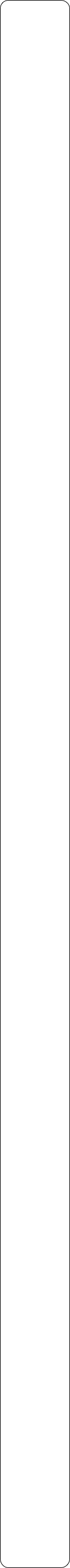Year 4 ObjectivesPlace ValuePre Tasks

(PitStops)

Main Tasks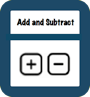Pre Tasks

(PitStops)

Main TasksMultiplication and Division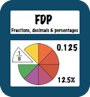Pre Tasks

(PitStops)

Main Tasks

Pre Tasks

(PitStops)

Main Tasks

Number - Fractions

Addition and Subtraction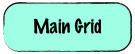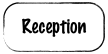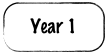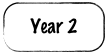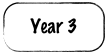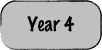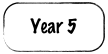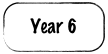- Count in multiples of 6, 7, 9, 25 and 1000- Find 1000 more or less than a given number

- Count backwards through zero to include negative numbers

- Recognise the place value of each digit in a four-digit number (thousands, hundreds, tens, and ones)

- Order and compare numbers beyond 1000

- Identify, represent and estimate numbers using different representations

- Round any number to the nearest 10, 100 or 1000

- Solve number and practical problems that involve all of the above and with increasingly large positive numbers

- Read Roman numerals to 100 (I to C) and know that over time, the numeral system changed to include the concept of zero and place value.- Add and subtract numbers with up to 4 digits using the formal written methods of columnar addition and subtraction where appropriate

- Estimate and use inverse operations to check answers to a calculation

- Solve addition and subtraction two-step problems in contexts, deciding which operations and methods to use and why.- Recall multiplication and division facts for multiplication tables up to 12 × 12

- Use place value, known and derived facts to multiply and divide mentally, including: multiplying by 0 and 1; dividing by 1; multiplying together three numbers

- Recognise and use factor pairs and commutativity in mental calculations

- Multiply two-digit and three-digit numbers by a one-digit number using formal written layout

- Solve problems involving multiplying and adding, including using the distributive law to multiply two digit numbers by one digit, integer scaling problems and harder correspondence problems such as n objects are connected to m objects.- Recognise and show, using diagrams, families of common equivalent fractions

- Count up and down in hundredths; recognise that hundredths arise when dividing an object by one hundred and dividing tenths by ten

- Solve problems involving increasingly harder fractions to calculate quantities, and fractions to divide quantities, including non-unit fractions where the answer is a whole number

- Add and subtract fractions with the same denominator

- Recognise and write decimal equivalents of any number of tenths or hundredths

- Recognise and write decimal equivalents to ¼, ½, ¾

- Find the effect of dividing a one- or two-digit number by 10 and 100, identifying the value of the digits in the answer as ones, tenths and hundredths

- Round decimals with one decimal place to the nearest whole number

- Compare numbers with the same number of decimal places up to two decimal places

- Solve simple measure and money problems involving fractions and decimals to two decimal placesSolutions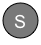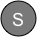StopsGap

StopsGap

StopsGap

StopsGap